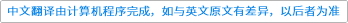Theoretical Computer Science ( IF 0.747 ) Pub Date : 2021-01-13 , DOI: 10.1016/j.tcs.2021.01.017
Baoling Ning; Jianzhong Li; Shouxu Jiang

Range partitioning is a typical and mostly used data partitioning method and has became a core operation in most of big data computing platforms. Given an input L of N data items admitting a total order, the goal of range partitioning is to divide the whole input into k ranges containing the same number of data items. There is a trivial lower bound $\mathrm{\Omega }\left(N\right)$ for the exact partitioning algorithms, since they need to at least make a full scan of the whole data. In the context of big data computing, even algorithms with $O\left(N\right)$ time are not always thought to be efficient enough, the ultimate goal of designing algorithms on big data is usually to solve problems within sublinear time. Therefore, it is well motivated and important to study sublinear algorithms for the range partitioning problem.

The paper aims to answer three questions. For the internal memory (RAM) model, since sophisticated sampling based $\left(ϵ,\delta \right)$-approximation partitioning algorithm with $O\left(\frac{k\mathrm{log}\left(N/\delta \right)}{{ϵ}^{2}}\right)$ time cost has been proposed, the first question is what a lower bound we can obtain for sublinear partitioning algorithms. For the external memory (I/O) model, as far as we know, no previous works give external partitioning algorithms with performance guarantee within sublinear time, therefore the two questions are what the upper bound and the lower bound we can achieve for sublinear external partitioning algorithms. To answer the above questions, based on the RAM and I/O model, the paper studies the lower and upper bounds for the range partitioning problem. For the RAM model, a lower bound $\mathrm{\Omega }\left(\frac{k\left(1-\delta \right)}{{ϵ}^{2}}\right)$ for the cost of sampling based partitioning algorithms is proved. For the I/O model, two lower bounds of the sampling cost required by sublinear external range partitioning algorithms are proved, which indicate that at least a full scan of the whole input is needed in the worst case and a general sublinear external partitioning algorithm does not exist. Motivated by the hard instances utilized in the proof of lower bounds, a model for describing the input distributions of the range partitioning problem in practical applications is proposed. Finally, for the special cases described by the model, a sublinear external partitioning algorithm with $O\left(\frac{k\mathrm{log}\left(N/\delta \right)}{wB{ϵ}^{2}}\right)$ I/O cost is designed.down
wechat
bug